Introducing algorithms

Years 3-4

Learning hook

Writing a sequence of instructions (algorithms) – whole class activity

1. Ask students to consider everyday tasks with a sequence of steps (for example, preparing to play a game using PlayStation, making a sandwich, fixing a puncture on a bicycle tyre, making a cake).

2. Ask students to suggest a sequence of steps for cleaning teeth. Remind them to sequence the steps in a logical order.

3. Share the Tellagami example with the class. Explain that this is a fast, easy and fun way to share information.

4. Rearrange the sequence of steps for cleaning teeth. Support students to understand why order is important when undertaking some tasks. Ask students if they can identify tasks where the order of instructions is very important.

Provide the class with the steps involved in making a vegemite sandwich (out of correct order).  Ask students to arrange the instructions in the correct order.

• Cut the sandwich in half
• Take two slices of bread from the packet
• Place the two slices of bread together

5. Give the class a list of everyday tasks. Ask students to select one task and independently write the steps necessary to complete the task.

Examples include:
• Make a vegemite sandwich
• Create and name a new folder on the desktop
• Make a paper plane
• Wash the dog
• Fix a bicycle tyre puncture
• Make a cup of Milo

Optional activity: (ICT capability)

Students may choose to share their sequence with others using a digital format. For example:
• Tellagami app
• short video
• short PowerPoint presentation
• concept mapping/ graphic organiser software
• infographic
• Voki

Learning map and outcomes

Graph paper programming

Explain that an algorithm is a list of steps that can be followed to complete a task.

Share the learning intentions for the lesson. For example:

Today we will …

design a sequence of steps (using words and symbols) for others to follow

evaluate the sequences of others to determine if the outcome was successful

Learning input

Dare to square game

1. Model the ‘Dare to square’ game.

2. Ask students to work in pairs. Provide students with a 4 x 4 grid with two black squares (Grid 1). Explain that the goal is to provide instructions to direct a partner to colour the specific squares within a grid.

For example:Example grid 1
Suggestion:

1. Move one square to the right.
2. Colour the square
3. Move one square to the right.
4. Move one square down.
5. Colour the square.
Or:
1. Move one square to the right.
2. Colour the square.
3. Move diagonally to the right.
4. Colour the square.

3. Provide each student with Example grid 2. Ask them to create a set of instructions to colour the correct squares.Example grid 2

4. After students complete the activity, invite them to share their instructions.

5. Display the instructions for all to view. For example:
1. Move one square to the right.
2. Colour the square.
3. Move one square to the right.
4. Move one square to the right.
5. Colour the square.
6. Move one square down.
7. Move one square to the left.
8. Colour the square.
9. Move one square to the left.
10. Move one square to the left.
11. Colour in the square.
12. Move one square down.
13. Move one square to the right.
14. Colour the square.
15. Move one square to the right.
16. Move one square to the right.
17. Colour the square.
18. Move one square down.
19. Move one square to the left.
20. Colour the square.
21. Move one square to the left.
22. Move one square to the left.
23. Colour the square.

6. Explain that these instructions would take a long time to write and even longer for a larger grid with more coloured squares.
Ask students to consider an easier and more effective way to provide the instructions. For example, could they use symbols?
Support the students to consider the use of arrows.

7. Provide the following suggestion:
 Move one square right Move one square left Move one square up Move one square down Colour the square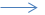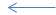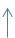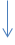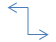*The program should look like this: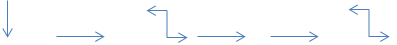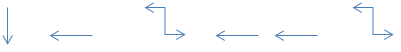Ask students to imagine they are providing the instructions to a computer. Tell them their task is to use the arrow symbols to provide the instructions instead of the words used previously.

Provide time for each student to use the arrow symbols to create a program instructing the computer to colour the correct squares on the grid.Learning demo

Think, pair share

1. Ask students to arrange themselves into pairs.

2. Provide each pair with Worksheet 1: Dare to square.

3. Explain that each pair will need to view the images on the worksheet. Using the arrow symbols, convert a sequence (algorithm) into a program for others to follow to ensure the correct squares on the grids are coloured.

4. Invite students to swap programs with other groups.

5. Provide each pair with blank grids and instruct the students to follow the programs to colour the squares on each grid.

6. Ask students to create their own versions of the grids and invite other students to provide instructions to complete the tasks.

7. Introduce the command repeat. Have the students consider how they can reduce the number of steps by including ‘repeat’ in the algorithm. For example, ‘move one square to the right, colour the square, repeat’.

Learning Consolidation

1.  Play ‘Rob the nest’
2. Working in pairs, one student is blindfolded and is required to follow a series of verbal instructions provided by his or her partner to retrieve an object (for example, a ball, toy or beanbag) from a set location (the ‘nest’).
3. Lead students to understand the importance of making sure instructions are clear and explicit enough to be followed. This could be a timed activity with the winning pair being those students who were able to retrieve the objects from the nest first or in the least amount of time.

Learning reflection

Encourage students to reflect on the task and share thoughts.
Highlight the positive behaviours observed during the lesson and revisit learning intentions.